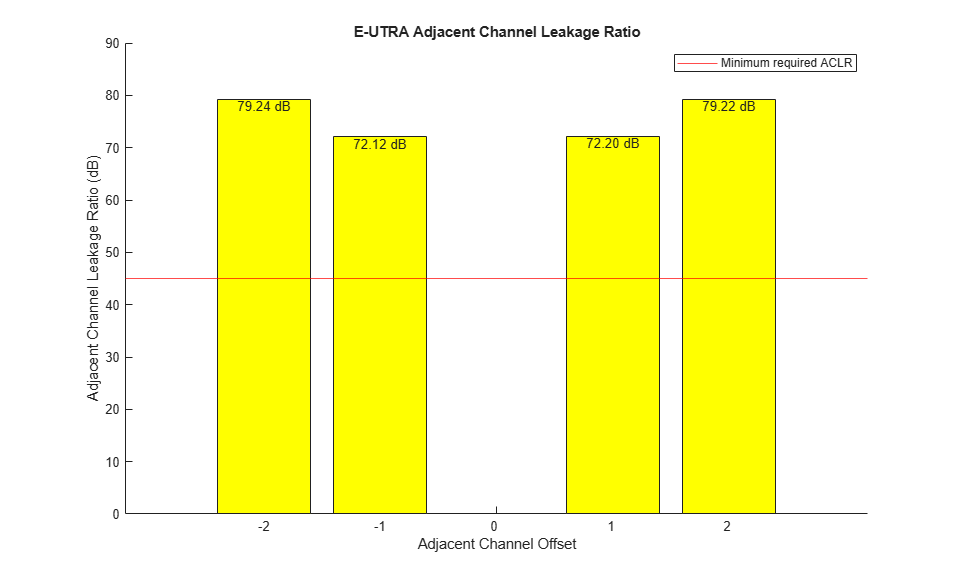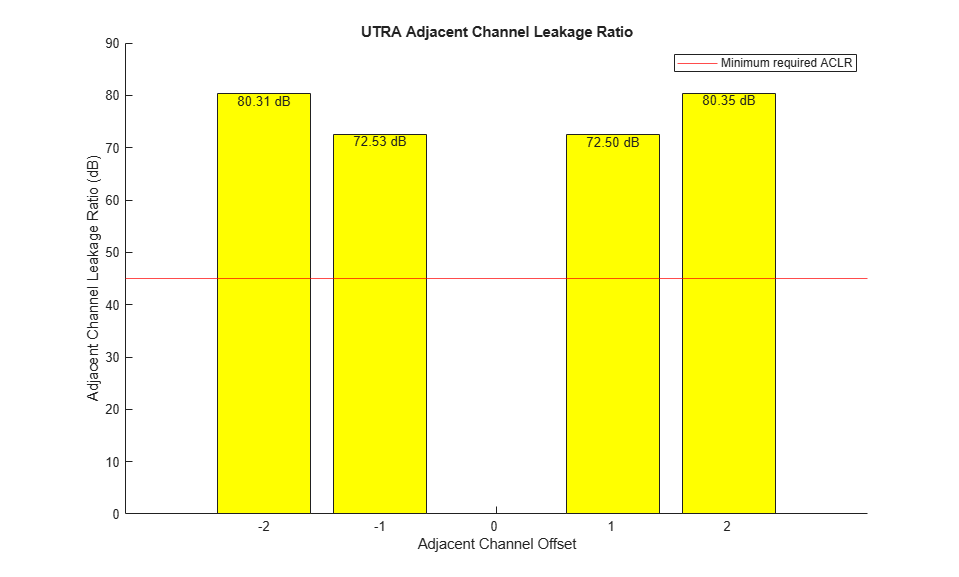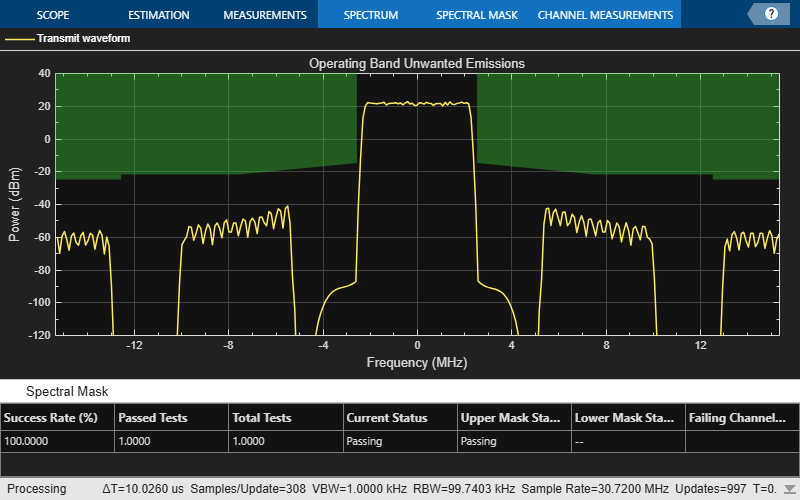Documentation

### This is machine translation

Mouseover text to see original. Click the button below to return to the English version of the page.

To view all translated materials including this page, select Country from the country navigator on the bottom of this page.

## LTE Downlink Adjacent Channel Leakage Power Ratio (ACLR) Measurement

This example shows how the Adjacent Channel Leakage Power Ratio (ACLR) can be measured within a downlink Reference Measurement Channel (RMC) signal using the LTE Toolbox™.

### Introduction

This example performs Adjacent Channel Leakage power Ratio (ACLR) measurements according to TS36.104, Section 6.6.2 [ 1 ] for a downlink waveform. ACLR is used as a measure of the amount of power leaking into adjacent channels and is defined as the ratio of the filtered mean power centered on the assigned channel frequency to the filtered mean power centered on an adjacent channel frequency. Minimum ACLR conformance requirements are given for E-UTRA (LTE) carriers and UTRA (W-CDMA) carriers.

The example is structured as follows:

• A downlink waveform is generated using a Reference Measurement Channel (RMC) configuration

• Parameters for ACLR measurements are calculated including the required oversampling rate to ensure a signal capable of representing both the E-UTRA and UTRA 1st and 2nd adjacent carriers with at most 85% bandwidth occupancy

• The waveform is oversampled as required

• The E-UTRA ACLR is calculated using a square measurement filter

• The UTRA ACLR is calculated using a Root Raise Cosine (RRC) filter

• The ACLR measurements are displayed

### Waveform Generation

A downlink RMC is used for measuring ACLR and created using `lteRMCDL` and `lteRMCDLTool`.

```% Generate the downlink configuration structure for RMC R.6 cfg = lteRMCDL('R.6'); % Generate the waveform which is a T-by-P matrix where T is the number of % time-domain samples and P is the number of receive antennas [waveform, ~, info] = lteRMCDLTool(cfg, [1; 0; 0; 1]); % Write the sampling rate and chip rate to the configuration structure to % allow the calculation of ACLR parameters cfg.SamplingRate = info.SamplingRate; cfg.UTRAChipRate = 3.84; % UTRA chip rate in MCPS ```

### Calculate ACLR Parameters

The parameters required for ACLR measurement are calculated using the helper function hACLRParameters.m.

• Determine Required Oversampling. If the input waveform sampling rate (`cfg.SamplingRate`) is not sufficient to span the entire bandwidth (`aclr.BandwidthACLR`) of the adjacent channels (allowing for a maximum of 85% bandwidth occupancy), an upsampled version of the waveform must be used for ACLR calculations. `aclr.OSR` is the upsampling factor.

• Determine UTRA Parameters; the chip rates and bandwidths.

```% Calculate ACLR measurement parameters [aclr, nRC, R_C, BWUTRA] = hACLRParameters(cfg); ```

### Perform Filtering of the Waveform to Improve ACLR

The waveform generated above has no filtering, so there are significant out of band spectral emissions owing to the implicit rectangular pulse shaping in the OFDM modulation (each OFDM subcarrier has a sinc shape in the frequency domain). In order to achieve a good ACLR performance, filtering must be applied to the waveform. A filter is designed with a transition band that starts at the edge of the occupied transmission bandwidth (`aclr.BandwidthConfig`) and stops at the edge of the overall channel bandwidth (`aclr.Bandwidth`). This filter involves no rate change, it just shapes the spectrum within the original bandwidth of the waveform. The filter is first designed, then applied to the waveform.

```% Design filter firFilter = dsp.LowpassFilter(); firFilter.SampleRate = info.SamplingRate; firFilter.PassbandFrequency = aclr.BandwidthConfig/2; firFilter.StopbandFrequency = aclr.Bandwidth/2; % Apply filter waveform = firFilter(waveform); ```

### Calculate E-UTRA and UTRA ACLR

The ACLR for E-UTRA and UTRA is measured using two helper functions:

• hACLRMeasurementEUTRA.m measures the E-UTRA ACLR using a square window on adjacent channels. A DFT of the measurement signal is taken and the energy of the appropriate bins used to calculate the adjacent channel powers.

• hACLRMeasurementUTRA.m measures the UTRA ACLR using a RRC filter on adjacent channels with a roll-off factor of 0.22 and bandwidth equal to the chip rate.

```% Apply required oversampling resampled = resample(waveform,aclr.OSR,1); % Calculate E-UTRA ACLR aclr = hACLRMeasurementEUTRA(aclr, resampled); % Calculate UTRA ACLR aclr = hACLRMeasurementUTRA(aclr, resampled, nRC, R_C, BWUTRA); ```

### Display Results

The ACLR results are returned in structure `aclr`. `aclr` contains the fields:

• `Bandwidth` : The channel bandwidth associated with `cfg.NDLRB` or `cfg.NULRB`, in Hertz. This is the overall bandwidth of the assigned channel.

• `BandwidthConfig` : The transmission bandwidth configuration associated with `cfg.NDLRB` or `cfg.NULRB`, in Hertz. This is the bandwidth within the channel bandwidth that contains active subcarriers.

• `BandwidthACLR` : The bandwidth required to represent both the E-UTRA and UTRA 1st and 2nd adjacent carriers; the sampling rate used internally for ACLR measurements will support this bandwidth with at most 85% bandwidth occupancy.

• `OSR` : The integer oversampling ratio of the input `waveform` required to create a signal capable of representing both the E-UTRA and UTRA 1st and 2nd adjacent carriers i.e. to represent `aclr.BandwidthACLR` with at most 85% bandwidth occupancy.

• `SamplingRate` : The sampling rate of the internal measurement signal from which the ACLR is calculated. If `OSR=1`, this signal is the input waveform; if `OSR>1`, this signal is the input waveform upsampled by `OSR`. Therefore: `aclr.SamplingRate = OSR*cfg.SamplingRate`.

• `EUTRAPowerdBm` : The power, in decibels relative to 1mW in 1ohm, of the input within the E-UTRA channel of interest i.e. in a square filter of bandwidth `aclr.BandwidthConfig` centered at 0Hz.

• `EUTRAdB` : A vector of E-UTRA ACLRs, in decibels relative to `aclr.EUTRAPowerdBm`, measured for adjacent channels [-2, -1, 1, 2].

• `EUTRACentreFreq` : A vector of E-UTRA center frequencies, in Hertz, for adjacent channels [-2, -1, 1, 2].

• `UTRAPowerdBm` : A vector of powers, in decibels relative to 1mW in 1ohm, of the input within the UTRA channel of interest; each element of the vector corresponds to each of the configured UTRA chip rates i.e. `UTRAPowerdBm(i)` gives the power of the input in an RRC filter designed for `R=cfg.UTRAChipRate(i)` Mchips/s, `alpha=0.22`, centered at 0 Hz.

• `UTRAdB` : A matrix of UTRA ACLRs, in decibels relative to `aclr.EUTRAPowerdBm`. The columns give the values for adjacent channels [-2, -1, 1, 2] and the rows give the values for each of the configured UTRA chip rates. Note that as required by the standard, these ACLRs are relative to `aclr.EUTRAPowerdBm`, not `aclr.UTRAPowerdBm`.

• `UTRACenterFreq` : A matrix of UTRA center frequencies, in Hertz. The columns give the values for adjacent channels [-2, -1, 1, 2] and the rows give the values for each of the configured UTRA chip rates.

hACLRResults.m displays the ACLR and plots the adjacent channel powers.

```hACLRResults(aclr); ```
``` Bandwidth: 5000000 BandwidthConfig: 4500000 BandwidthACLR: 25000000 OSR: 4 SamplingRate: 30720000 EUTRACenterFreq: [-10000000 -5000000 5000000 10000000] EUTRAPowerdBm: -0.5918 EUTRAdB: [79.2357 72.1187 72.2046 79.2157] UTRAPowerdBm: -1.3397 UTRAdB: [80.3117 72.5323 72.5011 80.3540] UTRACenterFreq: [-10000000 -5000000 5000000 10000000] ```### Operating Band Unwanted Emissions (Spectral Mask)

The waveform spectrum is displayed in conjunction with the transmit spectral mask defined in TS 36.104. This example assumes that the waveform corresponds to a Medium Range BS as described in TS 36.104 Table 6.2-1 and the power is set to 38.0dBm (~21.5dBm/100kHz). The corresponding spectral mask is TS 36.104 Table 6.6.3.2C-5 "Medium Range BS operating band unwanted emission limits for 5, 10, 15 and 20MHz channel bandwidth, P_max,c <= 31dBm".

```% Adjust waveform power to maximum rated output power P_max = 38.0; % TS 36.104 Table 6.2-1 bsWaveform = resampled * 10^((P_max-aclr.EUTRAPowerdBm)/20); % Create a spectrum analyzer, configure it for the waveform sampling rate % and a resolution bandwidth of 100kHz, configure and display the spectral % mask in TS 36.104 Table 6.6.3.2C-5, and perform spectrum analysis of the % waveform spectrumAnalyzer = dsp.SpectrumAnalyzer; spectrumAnalyzer.Name = 'Operating Band Unwanted Emissions'; spectrumAnalyzer.Title = spectrumAnalyzer.Name; spectrumAnalyzer.RBWSource = 'Property'; spectrumAnalyzer.RBW = 100e3; spectrumAnalyzer.SampleRate = info.SamplingRate * aclr.OSR; spectrumAnalyzer.ShowLegend = true; spectrumAnalyzer.ChannelNames = {'Transmit waveform'}; spectrumAnalyzer.YLimits = [-120 40]; % "Frequency offset of measurement filter centre frequency" in table f_offset = [0.05; 5.05; 10.05; 10.05] * 1e6; % "Minimimum requirement" in table (dBm/100kHz) mask_power = [(P_max-53); (P_max-60)*[1; 1]; min(P_max-60,-25)*[1; 1];]; % Add vertical mask segment at the band edge; no particular in-band power % mask is required to be met for this test f_offset = [repmat(f_offset(1),2,1); f_offset]; mask_power = [NaN; spectrumAnalyzer.YLimits(2); mask_power]; % Extend mask to analysis bandwidth edge, assumed to be closer to the edge % frequency of the carrier of interest than f_offset_max (the offset to the % frequency 10MHz outside the downlink operating band) mask_freq = [f_offset + aclr.Bandwidth/2; spectrumAnalyzer.SampleRate/2]; % Add a mirrored version of the mask to cover negative frequencies, and % enable the mask mask_power = [flipud(mask_power); mask_power]; mask_freq = [-flipud(mask_freq); (mask_freq)]; spectrumAnalyzer.SpectralMask.EnabledMasks = 'Upper'; spectrumAnalyzer.SpectralMask.UpperMask = [mask_freq, mask_power]; % Perform spectrum analysis spectrumAnalyzer(bsWaveform); ```### Perform Downsampling and Measure EVM

Finally the waveform is downsampled and resynchronized and an EVM measurement is performed. For more information on making EVM measurements, see PDSCH Error Vector Magnitude (EVM) Measurement.

```downsampled = resample(resampled,1,aclr.OSR); offset = lteDLFrameOffset(cfg,downsampled,'TestEVM'); cec.PilotAverage = 'TestEVM'; evmsettings.EnablePlotting = 'Off'; evm = hPDSCHEVM(cfg,cec,downsampled(1+offset:end,:),evmsettings); ```
```Low edge EVM, subframe 0: 0.739% High edge EVM, subframe 0: 0.705% Low edge EVM, subframe 1: 0.885% High edge EVM, subframe 1: 0.789% Low edge EVM, subframe 2: 0.785% High edge EVM, subframe 2: 0.699% Low edge EVM, subframe 3: 0.698% High edge EVM, subframe 3: 0.666% Low edge EVM, subframe 4: 0.817% High edge EVM, subframe 4: 0.640% Low edge EVM, subframe 6: 0.840% High edge EVM, subframe 6: 0.747% Low edge EVM, subframe 7: 0.732% High edge EVM, subframe 7: 0.696% Low edge EVM, subframe 8: 0.744% High edge EVM, subframe 8: 0.748% Averaged overall EVM: 0.783% ```

### Further Exploration

You can modify parts of this example to calculate ACLR (as per TS36.101, Section 6.6.2.3 [ 2 ]) for the uplink by using `lteRMCUL` in the place of `lteRMCDL` to generate the waveform.

### Appendix

This example uses the following helper functions:

### Selected Bibliography

1. 3GPP TS 36.104 "Base Station (BS) radio transmission and reception"

2. 3GPP TS 36.101 "User Equipment (UE) radio transmission and reception"